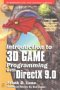# Maths - Vectors - forum discussion

 By: Nobody/Anonymous - nobodyDirection of cross product vector is wrong   2005-04-17 08:24 The direction of the cross product vector in this diagram is the opposite of what it should be. i.e. AxB points in the opposite direction.    https://www.euclideanspace.com/maths/algebra/vectors/crossProduct.gif
 By: Richard - redeyes2003RE: Direction of cross product vector is wrong   2005-04-17 23:56 Cross product is a mathematical convention where X, Y and Z are concerned    Given one conventional definition for the crossproduct x, we have  X = Y x Z  Y = Z x X  Z = X x Y    Drawing axes is also another convention, a pictorial one. If you use the crossproduct convention above (purely mathematical) with a pictorial convention similar to DirectX (the coordinate system is stated with y upwards, x to the right and z forward) then the mathematical convention must be represented by the pictorial convention, eg AxB = C and like X x Y= Z then C must be in the same picture representation as Z.    Note you can change the pictorial convention without changing the mathematical convetion. If OpenGl coordinate system is used then it is a mirror picture of the DirectX system, but C and Z still coincides.    Note you could change the mathematical convention  X = Z x Y  Y = X x Z  Z = Y x X  Here mathematically the result is different    Everything is ok when you stick to one convention throughout your representation :) I have not read through all of the context from  https://www.euclideanspace.com/maths/algebra/vectors/  but the diagram is just on element out of the entire context, so I cant agree or disagree with you
 By: Martin Baker - martinbakerRE: Direction of cross product vector is wron   2005-04-18 07:35 Thanks very much for the correction I have updated the page.    As Richard pointed out the problem was that I drew the diagram with a left hand coordinate system although I am standardising the site with a right hand coordinate system (for compatibly with OpenGL and X3D). I have redrawn the diagram with a right hand coordinate system and because it is difficult to represent the 3 dimensions I have added an example.    Although both right and left hand conventions are commonly used for the coordinate systems, does anyone know if it is common to use different conventions for cross products?    Thanks for your help,    Martinmetadata block see also: Correspondence about this page Book Shop - Further reading. Where I can, I have put links to Amazon for books that are relevant to the subject, click on the appropriate country flag to get more details of the book or to buy it from them.Introduction to 3D Game Programming with DirectX 9.0 - This is quite a small book but it has good concise information with subjects like, maths introduction and picking.Mathematics for 3D game Programming - Includes introduction to Vectors, Matrices, Transforms and Trigonometry. (But no euler angles or quaternions). Also includes ray tracing and some linear & rotational physics also collision detection (but not collision response). Other Math Books Specific to this page here:

This site may have errors. Don't use for critical systems.